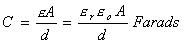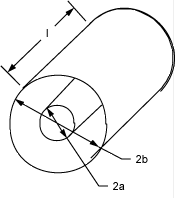# Capacitance Formulas

2 Parallel Plates
Coaxial Cylinders

The formulas on this page allow one to calculate the capacitance for certain given geometries.  More complex problems typically require one to model the geometry and solve using Finite Element Analysis.

2 Parallel Plane PlatesDiagram of a Parallel Plate CapacitorEquation for a Parallel Plate Capacitor e is the permittivity (F/m) e0 is the permittivity of free space (F/m) er is the relative permittivity of the dielectric A is the area of the electrodes (length, l * width, w in m2) d is the distance between the plates (m)

2 Coaxial CylindersDiagram for a Coaxial Cylinder CapacitorEquation for a Coaxial Cylinder Capacitor e is the permittivity (F/m) e0 is the permittivity of free space (F/m) er is the relative permittivity of the dielectric a is the radius of the inner cylinder b is the radius of the outer cylinder l is the length of the cylinders (m)

Send consulting inquiries, comments, and suggestions to richard.ness@nessengr.com